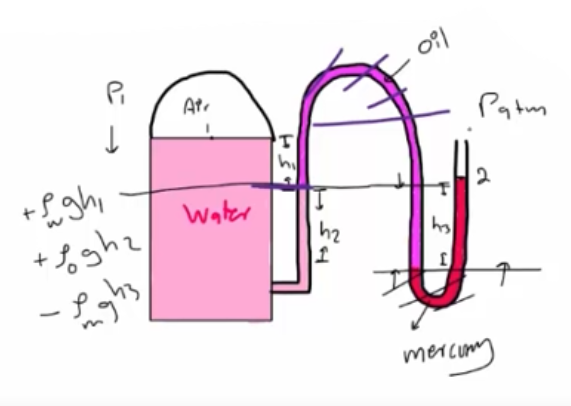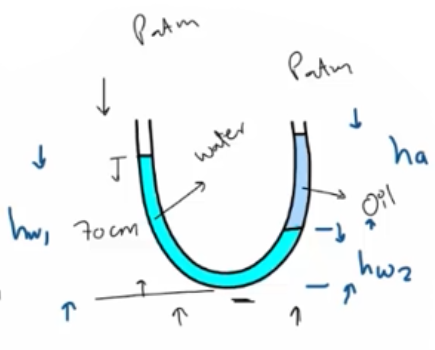Need Help?

Subscribe to Thermodynamics

###### \${selected_topic_name}
• Notes
• Comments & Questions

$\text { The water in a tank is pressurized by air, and the pressure is measured by a multifluid manometer }$

$\text { as shown in Fig. Pl-53. Determine the gage pressure of air in the tank if } l_{i,}^{\prime}=0.2 \mathrm{m}, \mathrm{h} 2=0.3 \mathrm{m}, \text { and }$

$h 3=0.46 \mathrm{m} . \text { Take the densities of water, oil, and mercury to be } 1000 \mathrm{kg} / \mathrm{m} 3,850 \mathrm{kg} / \mathrm{m} 3, \text { and } 13,600 \mathrm{kg} / \mathrm{m} 3, \text { respectively. }$$P_{1}+\rho_{w} g h_{1}+\rho_{0} g h_{2}$$-\rho g h_{3}=P_{atm}$

$P_{1}-P_{a t m}=$$\rho g h_{3}-\rho_{w} g h_{1}$$-\rho_0 \mathrm{gh}_{2}$

$P_{1} - P_{a t m}$$=g\left(\rho_{m} h_{3}-\rho_{w} h_{1}-\rho_{0} h_{2}\right)$

$\text{P}_ \text{gage }=P_{1}-P_{\text { atm }}$
$∴ \quad \text P_\text { 1gage }=g \left(\rho_m h_{3}-\rho_{w} h_{1}-\rho_{0} h_{2}\right)$

$=9.81(13600 *(0.46)-1000(0.2)-850(0.3))$

$=56.9 \mathrm{kPa}$

$\text { Consider a U-tube whose arms are open to the atmosphere. Now water is poured into }$

$\text { the U-tube from one arm, and light oil }(p=790 \mathrm{kg} / \mathrm{m} 3) \text { from the other. One arm }$

$\text { contains } 70 \text { -cm-high water, while the other arm contains both fluids with an }$

$\text { oil-to-water height ratio of } 4 . \text { Determine the height of each fluid in that arm. }$$\rho_{\text{ oil }}=790 \mathrm{kg} / \mathrm{m}^{3}$

$\rho_{w}=1000 \mathrm{kg} / \mathrm{m}^{3}$

$h_{a}=4 h_{w 2}=4(0.168)\left(\begin{array}{c}{0.673} {m}\end{array}\right)$

$P_{\text { bottom }}=P_\text { at m}+\rho_{w} g h w_{1}$

$P_\text {bottom = }$$P_\text { atm }$$+\rho_\text {w} ghw_ 2$$+\rho_{0} g h_{a}$

$\rho_ { w} ghw_1$$=\rho_{w} ghw_{2}+\rho_{a} g h a$

$\rho_{w} hw_{1}=\rho_{w} hw_{2}+\rho_{0} h_{a}$

$÷ \rho_w\rightarrow h w_{1}= \frac{\rho_{w} hw_{2}}{\rho_w}+\frac{\rho_{0} h a}{\rho_{w}}\quad\longrightarrow h{w_{1}}=h w_{2}+\frac{\rho_{0} h_{4}}{\rho_{w}}$

$\longrightarrow \quad 0.7=h w_{2}+$$\frac{790 \mathrm{ha}}{1000}$

$\longrightarrow \quad 0.7=h w_{2}+\frac{790hw_2 (4)}{1000}$

$\text { hw} _2=0.168$

No comments yet